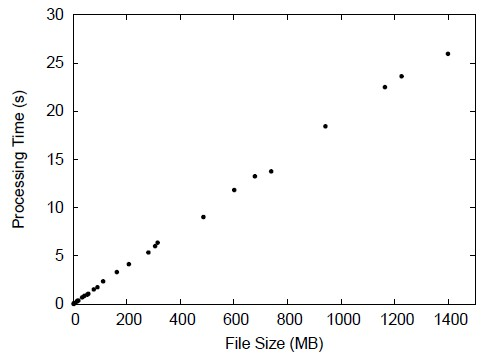Skip to content →

# Category: MD5 Analysis

A project to exam the computational complexity and security features of MD5. Done in 2009.

In this project, we explored the performance of the popular hash function MD5 — Message Digest Algorithm Version 5. Firstly we introduce the algorithm in detail. Then we check the algorithm’s complexity in two aspects: computational and space complexity. Furthermore we check the security aspects of MD5: whether it is feasible to find collision it using brute force, birthday attack, and cryptanalysis. Available cryptanalysis methods are introduced so that we can find out why MD5 is not considered secure any more.

The computational complexity of MD5 can be seen from the following diagram:The bash script used to generate the above diagram is as follows: